# Perpendicular line proofs

## Perpendicular Lines Theorems

When we're dealing with a pair of lines, three relationships are possible. The lines can be parallel, perpendicular, or neither. When lines are parallel, they will never intersect (touch/cross) because they have the same slope, and are therefore always the same distance apart (equidistant). When lines are perpendicular, they do intersect, and they intersect at a right angle. This is because perpendicular lines are said to have slopes that are "negative reciprocals" of each other, which we'll get into more later. Lastly, when a pair of lines have slopes that are neither identical nor negative reciprocals, this pair of lines is neither parallel nor perpendicular. Check out our lesson on relationships between lines and angles for more explanations.

This image below summarizes the difference between parallel and perpendicular lines: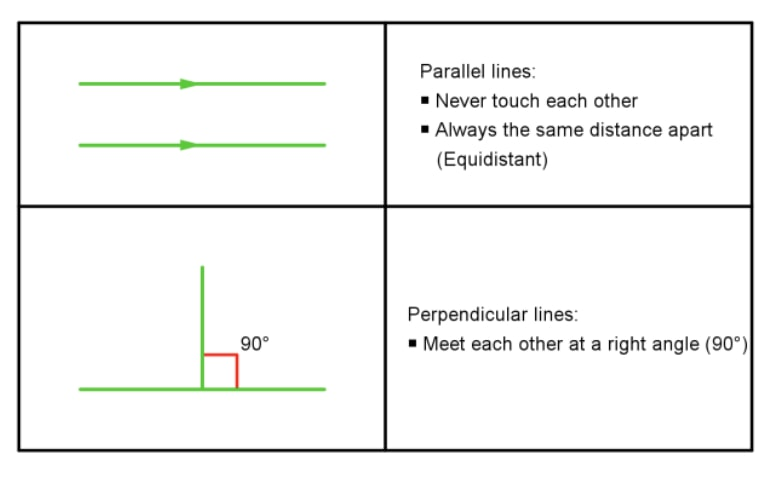The difference between parallel and perpendicular lines

Before you go further in this article, make sure you understand the difference between parallel and perpendicular lines.

Also, you may want to review the information on perpendicular bisector, which won't be covered in this article.

When dealing with perpendicular lines specifically, there are three general "theorems" that we can use to give us helpful information to solve more complex problems. Below are the three theorems, which we will be used later on in this article to make some proofs:

Theorem 1: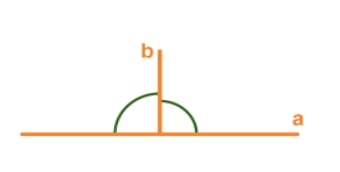Perpendicular when two lines intersect to form a pair of congruent angles

If two lines intersect to form a linear pair of "congruent angles", the lines are therefore perpendicular. Congruent angles are just angles that are equal to each other!

Theorem 2: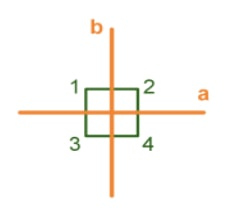Perpendicular when two lines intersect to form four right angles

If two lines are perpendicular, they will intersect to form four right angles.

Theorem 3: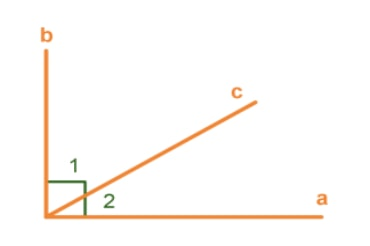Angles are complementary when two sides of two adjacent acute angles are perpendicular

If two sides of two "adjacent acute angles" are perpendicular, the angles are therefore complementary. Adjacent angles are angles that are beside each other, whereas acute angles, as you hopefully recall, are angles less then 90 degrees.

## How to Find Perpendicular Lines:

Now that we've defined what perpendicular lines are and what they look like, let's practice finding them in some practice problems.

Example 1:

In the image below, determine what set(s) of lines are perpendicular.Perpendicular line proofs with right angles

Step 1: Consider Lines r and Line p

Looking at the lines r and p, it is clear that they intersect at a right angle. Since this is the definition of perpendicular lines, line r is therefore perpendicular to line p.

Step 2: Consider Lines r and q

Looking at the lines r and q now, it is also apparent that they intersect at a right angle. Again, since this is the definition of perpendicular lines, line r is also perpendicular to line q.

Step 3: Consider Lines p and q

Lastly, let's take a look at the lines p and q. In the image, we can clearly see that lines p and q do not intersect, and will never intersect based on their slopes. Therefore, we can conclude that lines p and q are not perpendicular, but are instead parallel.

Example 2:

In the image below, determine what set(s) of lines are perpendicular.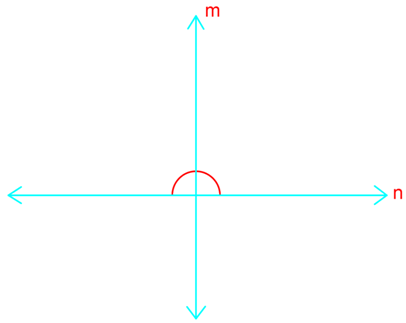Proving perpendicular line with right angles

Solving this problem is similar to the process in Example 1. Look at the angles formed at the intersection. Since the angles are congruent, leading to perpendicular angles, according to Theorem 1 discussed earlier, the lines m and n are therefore perpendicular.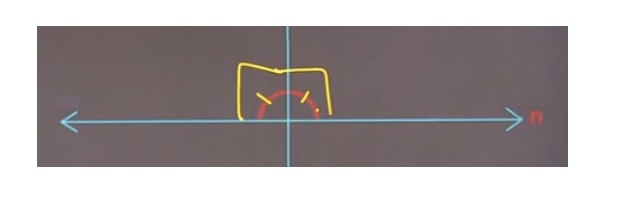The reason why m and n are perpendicular

Example 3:

In the image below, determine what set(s) of lines are perpendicular.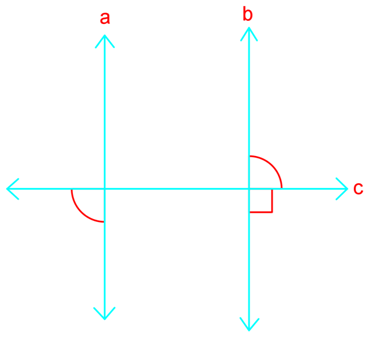Perpendicular line and 90 degree angles

Step 1: Consider Lines a and b

Let's take a look at lines a and b first. Clearly, as we have practiced in early examples, these two lines do not intersect, and are parallel, not perpendicular.

Step 2: Consider Lines b and c

Next, consider the lines b and c. From the image above, we can see that one of the angles formed between the lines' intersection is a 90 degree angle, and therefore, according to Theorem 2 discussed earlier, these lines are perpendicular.Line b and c perpendicular because of Theorem 2

Step 3: Consider Lines a and c

Lastly, let's look at the lines a and c. Because we know that the angle at the intersection of these two lines is congruent to one of the angles at the intersection of lines b and c, according to Theorem 1 discussed earlier, the lines a and c are therefore perpendicular.

## How to Prove Perpendicular Lines

In some problems, you may be asked to not only find which sets of lines are perpendicular, but also to be able to prove why they are indeed perpendicular. The best way to get practice proving that a pair of lines are perpendicular is by going through an example problem.

Example:

Write a proof for the following scenario:

Given that line m is perpendicular to line n, prove: that angle 1 and angle 2 are complementary to each other.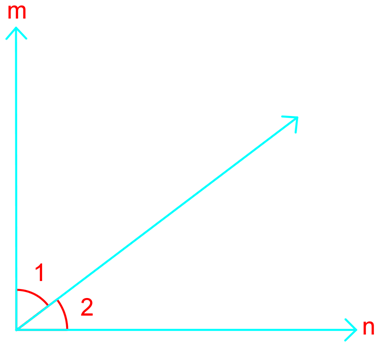Perpendicular line proofs

To prove this scenario, the best option is to take a look at the three theorems we discussed at the beginning of this article. If you recall, Theorem 3 states that "if two sides of two 'adjacent acute angles' are perpendicular, the angles are therefore complementary." In this scenario, we do indeed have a perpendicular angle formed by the lines m and n. This angle is split by the third diagonal line, which creates two adjacent acute angles – in accordance with Theorem 3. Therefore, using Theorem 3, we can successfully prove that angle 1 and angle 2 are complementary.

And that's all there is to it! For more information on parallel and perpendicular lines, and for some more practice problems, check out this helpful link here.

For further study into perpendicular and parallel lines, and for information regarding equations of lines, you can go to the sections on parallel and perpendicular lines in linear functions, perpendicular line equation, and combination of parallel and perpendicular line equations questions.

### Perpendicular line proofs

#### Lessons

• Introduction
Relationships between lines and angles

• 1.
Determine what set(s) of lines are perpendicular.
a)b)c)• 2.
Write a proof.
Given: $m \perp$ $n$
Prove: $\angle 1$ and $\angle 2$ are complementary.